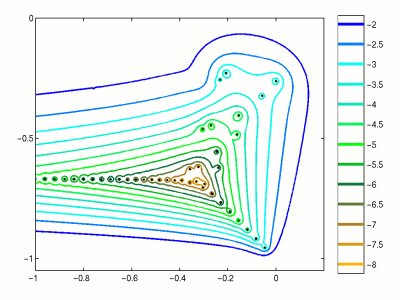Home | Introduction | Software | Examples | History | People | Bibliography | Applications | Theorems

## Pseudospectra of a Spectral Approximation of an Orr-Sommerfeld OperatorThis figure illustrates pseudospectra of an Orr-Sommerfeld operator generated from a spectral method. This discretization results in a matrix of dimension N=99. There are numerous spurious eigenvalues beyond the plotted axis, so the matrix is projected onto an appropriate invariant subspace to accelerate the computation. Pseudospectra of Orr-Sommerfeld operators were first investigated by Reddy, Schmidt, and Henningson [RSH93].

Use the following MATLAB code compute a similar image using EigTool.

```  R = 5772;
N = 100;
[D,x] = cheb(N);     % cheb.m from Trefethen's "Spectral Methods in MATLAB"
D2 = D^2; D2 = D2(2:N,2:N);
S = diag([0; 1 ./(1-x(2:N).^2); 0]);
D4 = (diag(1-x.^2)*D^4 - 8*diag(x)*D^3 - 12*D^2)*S;
D4 = D4(2:N,2:N);
I = eye(N-1);
A = (D4-2*D2+I)/R - 2i*I - 1i*diag(1-x(2:N).^2)*(D2-I);
B = D2-I;
C = B\A;
opts.npts=50;
opts.ax = [-1 .2 -1.05 0];
opts.levels = -10:.5:-2;
opts.proj_lev = 1;
eigtool(C,opts)
```
See also J. A. C. Weideman and S. C. Reddy, "A MATLAB differentiation matrix suite", ACM Transactions on Math. Software, to appear, and program `p40.m` in L. N. Trefethen, Spectral Methods in MATLAB, SIAM, Philadelphia, 2000.